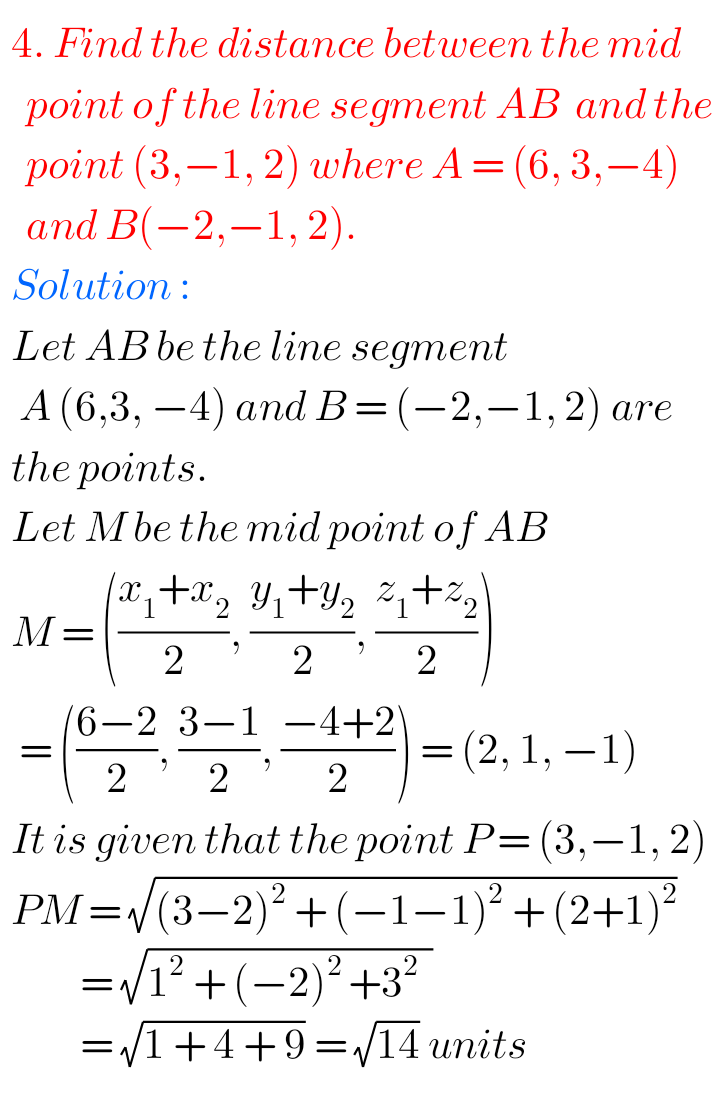## Inter maths solutions for Three Dimensional Coordinates exercise 5(b)

Three Dimensional Coordinates solutions Inter exercise 5(b) Intermediate Mathematics 1B Chapter 5 Three Dimensional Coordinates Exercise 5(b) textbook solutions are given. First you sytudy the textbook lesson very well. Then observe the example problems and solutions given in the textbook. The key points in the lesson are very important. Observe the solutions given below and …